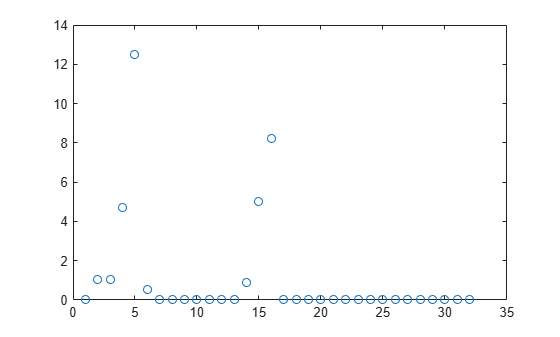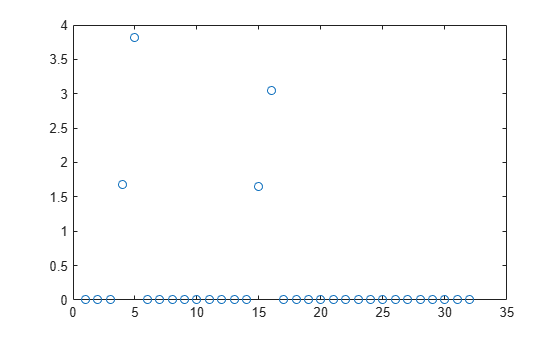# refit

Refit neighborhood component analysis (NCA) model for regression

## Syntax

```mdlrefit = refit(mdl,Name,Value) ```

## Description

`mdlrefit = refit(mdl,Name,Value)` refits the model `mdl`, with modified parameters specified by one or more `Name,Value` pair arguments.

## Input Arguments

expand all

Neighborhood component analysis model or classification, specified as a `FeatureSelectionNCARegression` object.

### Name-Value Arguments

Specify optional comma-separated pairs of `Name,Value` arguments. `Name` is the argument name and `Value` is the corresponding value. `Name` must appear inside quotes. You can specify several name and value pair arguments in any order as `Name1,Value1,...,NameN,ValueN`.

Fitting Options

expand all

Method for fitting the model, specified as the comma-separated pair consisting of `'FitMethod'` and one of the following.

• `'exact'` — Performs fitting using all of the data.

• `'none'` — No fitting. Use this option to evaluate the generalization error of the NCA model using the initial feature weights supplied in the call to `fsrnca`.

• `'average'` — The function divides the data into partitions (subsets), fits each partition using the `exact` method, and returns the average of the feature weights. You can specify the number of partitions using the `NumPartitions` name-value pair argument.

Example: `'FitMethod','none'`

Regularization parameter, specified as the comma-separated pair consisting of `'Lambda'` and a non-negative scalar value.

For n observations, the best `Lambda` value that minimizes the generalization error of the NCA model is expected to be a multiple of 1/n

Example: `'Lambda',0.01`

Data Types: `double` | `single`

Solver type for estimating feature weights, specified as the comma-separated pair consisting of `'Solver'` and one of the following.

• `'lbfgs'` — Limited memory BFGS (Broyden-Fletcher-Goldfarb-Shanno) algorithm (LBFGS algorithm)

• `'sgd'` — Stochastic gradient descent

• `'minibatch-lbfgs'` — Stochastic gradient descent with LBFGS algorithm applied to mini-batches

Example: `'solver','minibatch-lbfgs'`

Initial feature weights, specified as the comma-separated pair consisting of `'InitialFeatureWeights'` and a p-by-1 vector of real positive scalar values.

Data Types: `double` | `single`

Indicator for verbosity level for the convergence summary display, specified as the comma-separated pair consisting of `'Verbose'` and one of the following.

• 0 — No convergence summary

• 1 — Convergence summary including iteration number, norm of the gradient, and objective function value.

• >1 — More convergence information depending on the fitting algorithm

When using solver `'minibatch-lbfgs'` and verbosity level >1, the convergence information includes iteration log from intermediate minibatch LBFGS fits.

Example: `'Verbose',2`

Data Types: `double` | `single`

LBFGS or Mini-Batch LBFGS Options

expand all

Relative convergence tolerance on the gradient norm for solver `lbfgs`, specified as the comma-separated pair consisting of `'GradientTolerance'` and a positive real scalar value.

Example: `'GradientTolerance',0.00001`

Data Types: `double` | `single`

SGD or Mini-Batch LBFGS Options

expand all

Initial learning rate for solver `sgd`, specified as the comma-separated pair consisting of `'InitialLearningRate'` and a positive scalar value.

When using solver type `'sgd'`, the learning rate decays over iterations starting with the value specified for `'InitialLearningRate'`.

Example: `'InitialLearningRate',0.8`

Data Types: `double` | `single`

Maximum number of passes for solver `'sgd'` (stochastic gradient descent), specified as the comma-separated pair consisting of `'PassLimit'` and a positive integer. Every pass processes `size(mdl.X,1)` observations.

Example: `'PassLimit',10`

Data Types: `double` | `single`

SGD or LBFGS or Mini-Batch LBFGS Options

expand all

Maximum number of iterations, specified as the comma-separated pair consisting of `'IterationLimit'` and a positive integer.

Example: `'IterationLimit',250`

Data Types: `double` | `single`

## Output Arguments

expand all

Neighborhood component analysis model or classification, returned as a `FeatureSelectionNCARegression` object. You can either save the results as a new model or update the existing model as ```mdl = refit(mdl,Name,Value)```.

## Examples

expand all

`load('robotarm.mat')`

The `robotarm` (pumadyn32nm) dataset is created using a robot arm simulator with 7168 training and 1024 test observations with 32 features , . This is a preprocessed version of the original data set. Data are preprocessed by subtracting off a linear regression fit followed by normalization of all features to unit variance.

Compute the generalization error without feature selection.

```nca = fsrnca(Xtrain,ytrain,'FitMethod','none','Standardize',1); L = loss(nca,Xtest,ytest)```
```L = 0.9017 ```

Now, refit the model and compute the prediction loss with feature selection, with $\lambda$ = 0 (no regularization term) and compare to the previous loss value, to determine feature selection seems necessary for this problem. For the settings that you do not change, `refit` uses the settings of the initial model `nca`. For example, it uses the feature weights found in `nca` as the initial feature weights.

```nca2 = refit(nca,'FitMethod','exact','Lambda',0); L2 = loss(nca2,Xtest,ytest)```
```L2 = 0.1088 ```

The decrease in the loss suggests that feature selection is necessary.

Plot the feature weights.

```figure() plot(nca2.FeatureWeights,'ro')```Tuning the regularization parameter usually improves the results. Suppose that, after tuning $\lambda$ using cross-validation as in Tune Regularization Parameter in NCA for Regression, the best $\lambda$ value found is 0.0035. Refit the `nca` model using this $\lambda$ value and stochastic gradient descent as the solver. Compute the prediction loss.

```nca3 = refit(nca2,'FitMethod','exact','Lambda',0.0035,... 'Solver','sgd'); L3 = loss(nca3,Xtest,ytest)```
```L3 = 0.0573 ```

Plot the feature weights.

```figure() plot(nca3.FeatureWeights,'ro')```After tuning the regularization parameter, the loss decreased even more and the software identified four of the features as relevant.

References

 Rasmussen, C. E., R. M. Neal, G. E. Hinton, D. van Camp, M. Revow, Z. Ghahramani, R. Kustra, and R. Tibshirani. The DELVE Manual, 1996, http://mlg.eng.cam.ac.uk/pub/pdf/RasNeaHinetal96.pdf